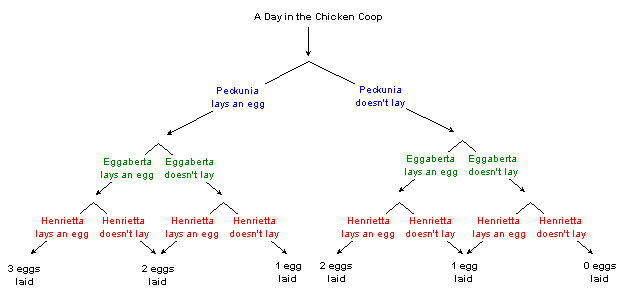SEARCH HOMEMath Central Quandaries & QueriesQuestion from joan, a teacher: A farmer has 3 hens. It is determined that each hen lays 6 eggs in one week. a)find the probability that a hen lays an egg in a randomly chosen day b) find the probability that each hen lays an egg in a randomly chosen day. c) find the probability that no hen lays an egg in a randomly chosen day. d) draw a tree diagram to illustrate the number of hens laying an egg in a randomly chosen day..Hi Joan.

1. I think the question means a specific hen. The chance that Henrietta the Hen will lay on a randomly chosen day of the week is 6 chances out of 7.

2. If we randomly choose a day, then we look at the chances that Henrietta and her two coop-mates (Eggaberta and Peckunia), then each has a 6/7 chance of laying (see part (a)). Therefore the chance that all three lay an egg that day is 6/7 x 6/7 x 6/7.

3. Peckunia's chance of laying an egg on a particular day is 6/7, so her chance of not laying an egg is 1/7. The chance that all three hens take the day off is therefore 1/7 x 1/7 x 1/7.

4.Cheers,
Stephen La Rocque.Math Central is supported by the University of Regina and The Pacific Institute for the Mathematical Sciences.